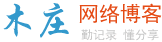# php遍历目录

```<?php
if(isset(\$_GET['id']))//判断是否传值
{
\$s=str_replace(' ','+',\$_GET['id']);
\$s=base64_decode(\$s);//接受传递的值 值一般是要打开的目录的绝对路劲
chdir(\$s);//切换到要打开的目录
}
\$a=opendir('.');//打开当前目录
{
if(is_dir(\$c))//判断是目录还是文件
{
if(\$c=='.')
{
}
else
{
if(\$c=='..')
{
\$w=base64_encode(substr(getcwd(),0,strrpos(getcwd(),'\\')));//当前路径加上目录名 就是目录的绝对路径
echo "上一级"."<a href='6.php?id=\$w'>打开</a><br />";//当点击打开时将要打开的绝对路径传递出去  用chdir 切换到要打开的目录下
}else
{
\$w=base64_encode(getcwd().'\\'.\$c);//当前路径加上目录名 就是目录的绝对路径
echo "\$c"."<a href='6.php?id=\$w'>打开</a><br />";//当点击打开时将要打开的绝对路径传递出去  用chdir 切换到要打开的目录下
}
}
}
else
{
echo "\$c 不是目录<br />";
}
}
?>

```

isset（）判断某个变量是否定义。

chdir（） 将当前目录改变为指定的目录。

opendir（） 打开目录。

getcwd()。获取当前目录。### 评论

• 欢迎访问木庄网络博客
• 可复制：代码框内的文字。
• 方法：Ctrl+C。